Courses

# NCERT Solution - Chapter 1 : Financial Statements - I (Part-1), Class 11, commerce | EduRev Notes

## Commerce : NCERT Solution - Chapter 1 : Financial Statements - I (Part-1), Class 11, commerce | EduRev Notes

The document NCERT Solution - Chapter 1 : Financial Statements - I (Part-1), Class 11, commerce | EduRev Notes is a part of the Commerce Course Accountancy Class 11.
All you need of Commerce at this link: Commerce

Page Number : 376

Numerical questions
Question.1 : From the following balances taken from the books of Simmi and Vimmi Ltd. for the year ending March 31, 2003, calculate the gross profit.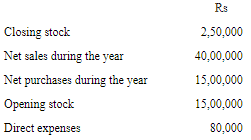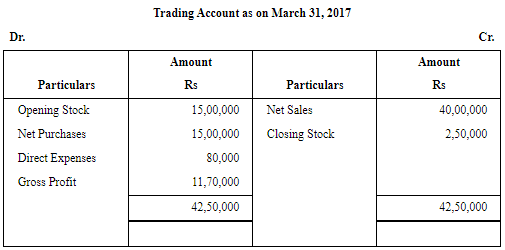Question.2 : From the following balances extracted from the books of M/s Ahuja and Nanda. Calculate the amount of:
(a) Cost of goods available for sale
(b) Cost of goods sold during the year
(c) Gross Profit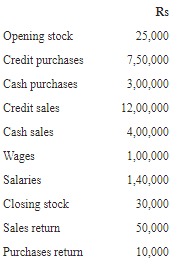(a) Cost of Goods Sold Available for Sales
Or
Cost of Goods Manufactured = Opening Stock + Net Purchases + Wages
= 25,000 + 10,40,000 + 1,00,000
= Rs 11,65,000
(b) Cost of Goods Sold = Opening Stock + Net Purchases + Wages - Closing Stock
= 25,000 + 10,40,000 + 1,00,000 - 30,000
= Rs 11,35,000
Or
Cost of Goods Sold = Net Sales - Gross Profit
= 15,50,000 - 4,15,000
= Rs 11,35,000

(c)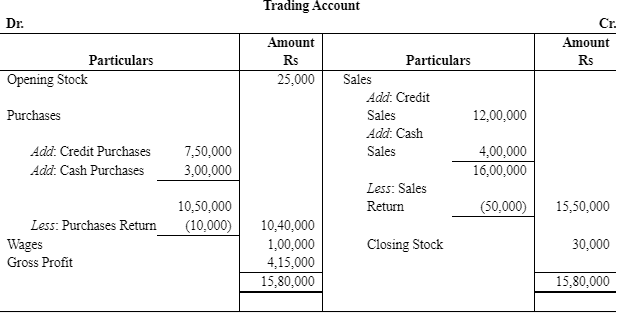Gross Profit Rs 4,15,000

Question.3 : Calculate the amount of gross profit and operating profit on the basis of the following balances extracted from the books of M/s Rajiv and Sons for the year ended March 31, 2005.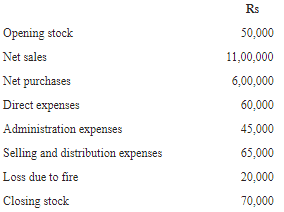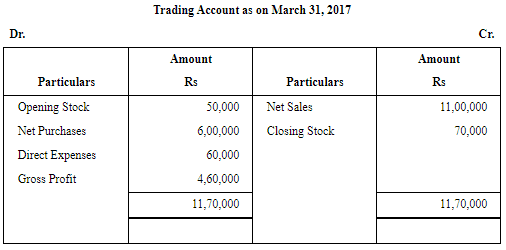Operating Profit = Sales - (Opening Stock + Net Purchases + Direct Expenses + Administration Expenses + Selling and Distribution Expenses) + Closing Stock
= 11,00,000 - (50,000 + 6,00,000 + 60,000 + 45,000 + 65,000) + 70,000
= Rs 3,50,000

Question.4 :  Operating profit earned by M/s Arora and Sachdeva in 2005-06 was Rs 17,00,000. Its non operating incomes were Rs 1,50,000 and non-operating expenses were Rs 3,75,000.  Calculate the amount of net profit earned by the firm.
Answer : Net Profit = Operating Profit + Non-operating Income - Non-operating Expenses
= 17,00,000 + 1,50,000 - 3,75,000
= Rs 14,75,000
Net profit earned by M/S Arora and Sachdeva in 2005-06 is Rs 14,75,000.

Question.5 : The following are the extracts from the trial balance of M/s Bhola and Sons as on March 31, 2005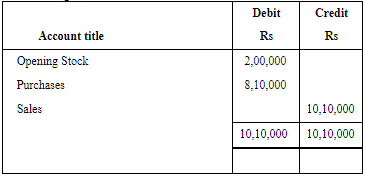(Only relevant items)
Closing Stock as on date was valued at Rs 3,00,000.
You are required to record the necessary journal entries and show how the above items will appear in the trading and profit and loss account and balance sheet of M/s Bhola and Sons.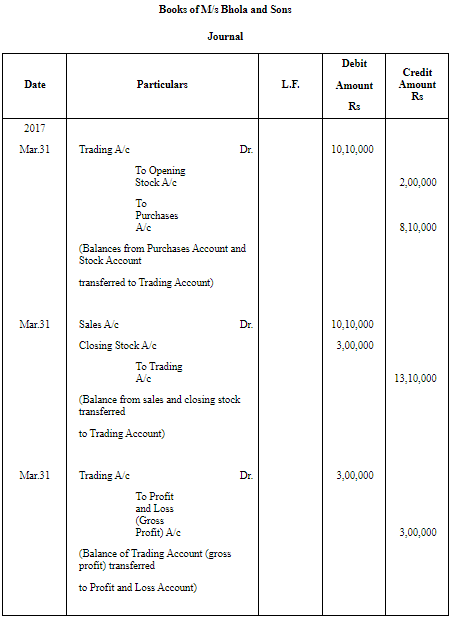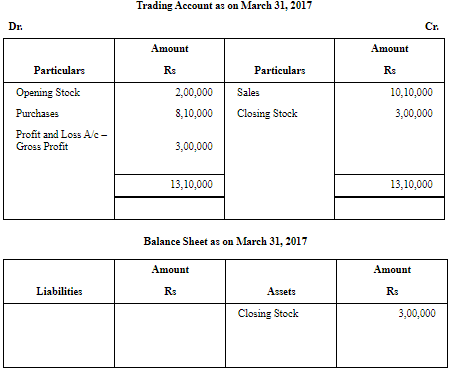Question.6 : Prepare trading and profit and loss account and balance sheet, as on March 31, 2017 :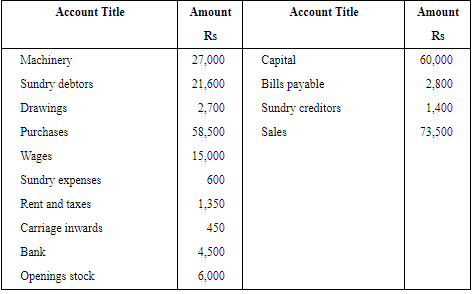Closing stock, as on March 31, 2017 Rs 22,400.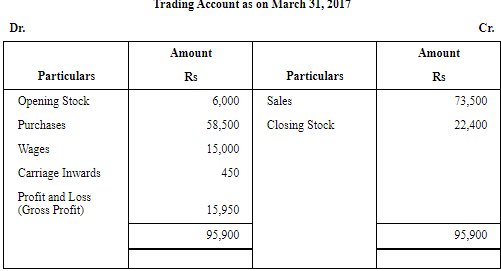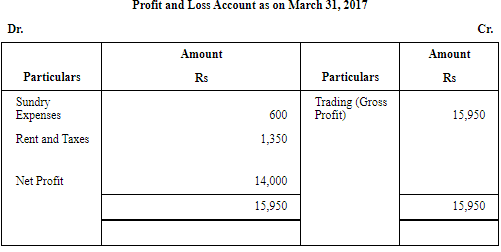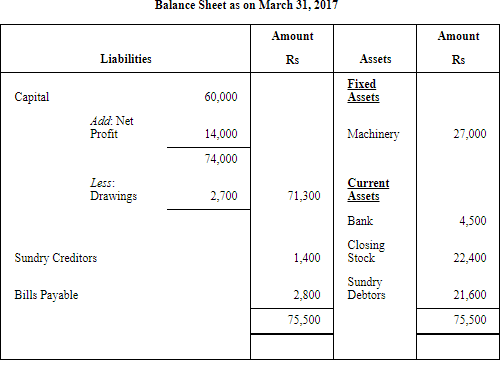Question.7 : The following trial balance is extracted from the books of M/s Ram on March 31, 2017. You are required to prepare trading and profit and loss account and the balance sheet as on date: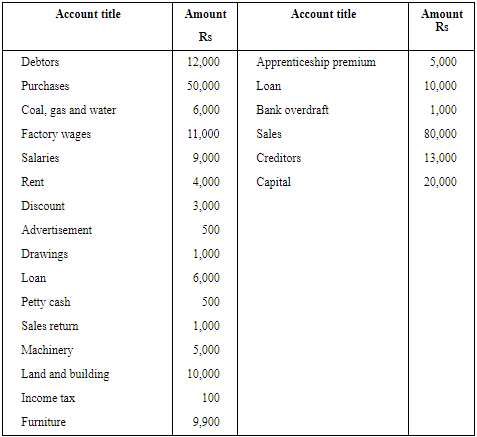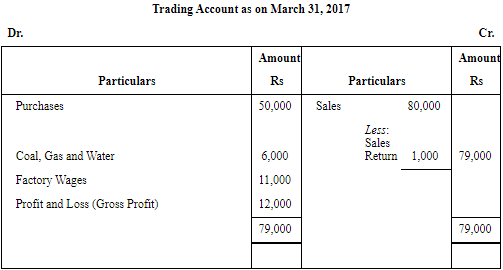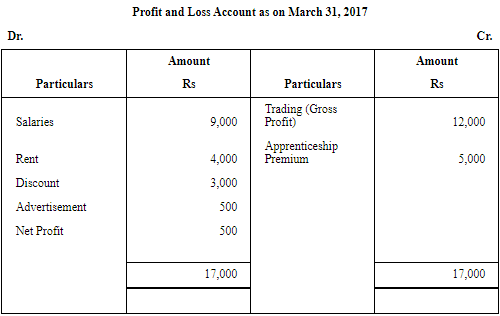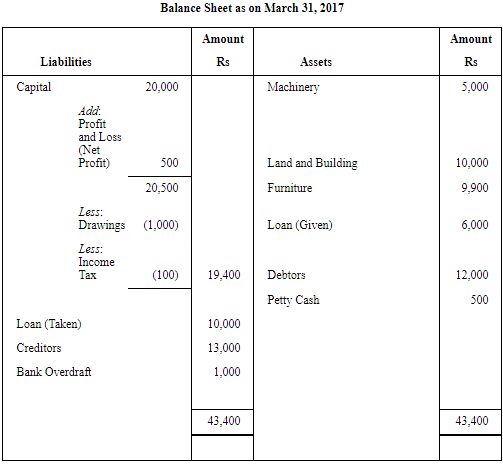Question.8 : The following is the trial balance of Manju Chawla on March 31, 2017. You are required to prepare trading and profit and loss account and a balance sheet as on date: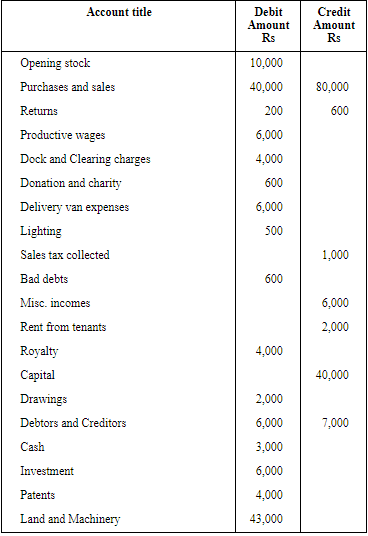Closing stock Rs 2,000.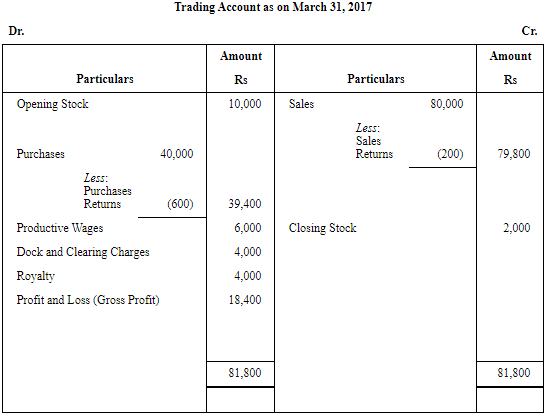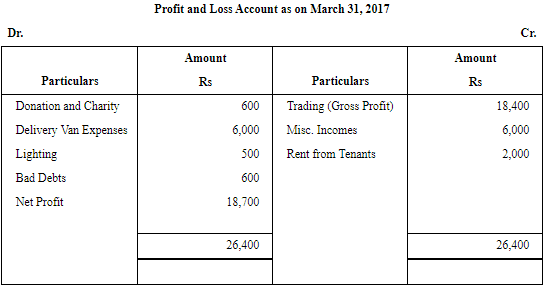Note: As per the solution, net profit is Rs 18,700; however, according to the answer given in the book, it is Rs 18,400.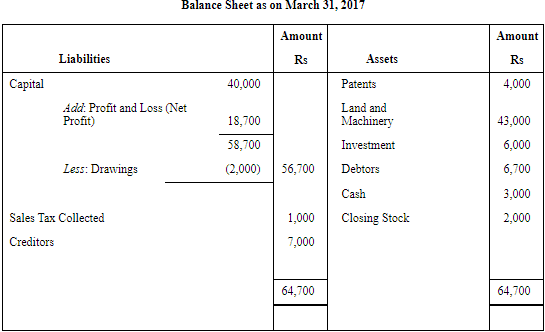Note: There is a misprint in the trial balance given in the question. In order to match the trial balance, debtors have been taken as Rs 6,700; however, the debtors given in the trial balance is Rs 60,000.

Offer running on EduRev: Apply code STAYHOME200 to get INR 200 off on our premium plan EduRev Infinity!

,

,

,

,

,

,

,

,

,

,

,

,

,

,

,

,

,

,

,

,

,

,

,

,

,

,

,

;